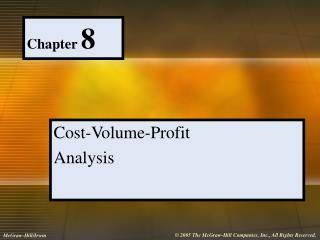Download PresentationChapter 8

# Chapter 8 - PowerPoint PPT Presentation

Chapter 8. Cost-Volume-Profit Analysis. What is Cost-Volume-Profit (CVP) Analysis?. Summarizes the effects of changes in an enterprise's volume of activity on its costs, revenue and profit. Definitions Match. Breakeven Point. Unit Contribution Margin / Unit Sales Price.I am the owner, or an agent authorized to act on behalf of the owner, of the copyrighted work described.
Download Presentation## Chapter 8

An Image/Link below is provided (as is) to download presentation

Download Policy: Content on the Website is provided to you AS IS for your information and personal use and may not be sold / licensed / shared on other websites without getting consent from its author.While downloading, if for some reason you are not able to download a presentation, the publisher may have deleted the file from their server.

- - - - - - - - - - - - - - - - - - - - - - - - - - E N D - - - - - - - - - - - - - - - - - - - - - - - - - -
Presentation Transcript
1. Chapter 8 Cost-Volume-Profit Analysis

2. What is Cost-Volume-Profit (CVP) Analysis? Summarizes the effects of changes in an enterprise's volume of activity on its costs, revenue and profit

3. Definitions Match Breakeven Point Unit Contribution Margin / Unit Sales Price Total Contribution Margin Unit Sales Price - Unit Variable Cost Unit Contribution Margin Total Sales - Total Variable Costs Contribution Margin Ratio Revenues = Expenses

4. Break-even in Dollars = Fixed Expenses Unit Contribution Margin Unit Sales Price OR Break-even in Dollars Fixed Expenses Contribution Margin Ratio = Break-even in Units Fixed Expenses Contribution Margin = What Are the Formulas for Breakeven in Dollars & Units?

5. \$ Break-Even Point Revenue Total Cost Variable Fixed LOSS Profit Activity Prepare & Label a CVP Graph

6. Break-Even Point Contribution Margin Profit Fixed Costs Loss Prepare & Label a Volume Profit Graph \$ Activity

7. Work Out the Equation Approach for a Target Profit Where: U = sales volume in units, SP = sales price of one unit, VC = variable cost of one unit, FC = total fixed costs, CM = contribution margin & TP = target profit (SP X U) – (VC X U) – FC = TP ((SP– VC) X U) – FC = TP Hint: SP – VC is the definition of CM (CM X U) – FC = TP The result is number of units that need to be sold to obtain a specified target profit U = TP + FC CM

8. Define Safety Margin The difference between budgeted sales revenue and break-even sales revenue or how much in sales can be lost and still break-even.

9. Comment on Applying CVP to • Changes in Fixed Expenses • Donations to Offset Fixed Expenses • Changes in Unit Contribution Margin • Changes in Unit Variable Costs • Changes in Sales Price

10. Given: Fixed Expenses Unit Contribution Margin Target Net Profit Find: Required Sales Volume Given: Fixed Expenses Unit Contribution Margin Expected Sales Volume Find: Target Net Profit What Can Be Determined From CVP Analysis?

11. Discuss the Incremental Approach • Simpler • More Depth of Understanding Required • Doesn’t Examine Whole Income Statement • Focuses on Difference in the Total Contribution Margin Under the Two Prices

12. Definitions Match Sales Mix Technique for determining what happens if a key assumption is in error Weighted-Average Contribution Margin per Unit Relative proportion of sales mix for each of an organization’s products Average of several product’s unit cm weighted by proportion it is of total sales Sensitivity Analysis

13. What is Different About CVP With Multiple Products? • The denominator is the “weighted-average contribution margin per unit.” • Assume sales mix cannot & will not change. • Can examine the change in sales mix, in addition to changes in sales volume, prices, variable costs & fixed costs.

14. List & Explain Assumptions of CVP Analysis • Total revenue is linear. • Total expenses are linear. • Can categorize expenses as fixed or variable. • Total fixed expenses are constant in relevant range. • Unit variable cost remains constant per unit as activity changes. • Efficiency & productivity are constant. • Sales mix constant for multiple products. • Inventory levels constant, sell all made.

15. Set up a Simple Traditional Income Statement Company Name Income Statement Date Sales Less: Cost of Goods Sold Gross Margin Less: Operating Expenses Selling Expenses Administrative Expenses Net Income

16. Setup a Contribution Margin Income Statement Same Heading Sales Less: Variable Expenses Variable Manufacturing Variable Selling Variable Administrative Contribution Margin Less: Fixed Expenses Fixed Manufacturing Fixed Selling Fixed Administrative Net Income

17. What is Cost Structure & Operating Leverage? • Cost structure is the relative proportion of its fixed costs versus variable costs. Or, what percent of the costs are fixed & what percent are variable. • Operating leverage is the extent that an organization uses its fixed costs. Higher proportion of fixed costs means higher operating leverage.

18. Contribution Margin Net Income Operating Leverage Factor = What Does Operating Leverage Mean & How Do You Measure It? • Operating leverage is the ability to generate excess profits from increases in sales. Because fixed costs are fixed, additional sales contribute more to profits when they have a larger portion of fixed costs versus variable costs.

19. How Does ABC Affect CVP Analysis? • ABC provides a more complete picture of cost-volume-profit relationships. • ABC provides better information to managers.

20. Comment on the Impact of JIT & Flexible Manufacturing on CVP • Variable costs should be lower • Fixed costs will be greater • Quicker setups • More frequent production runs • Fewer inspections • Greater depreciation expense

21. Chapter 8 Is Complete